# Time value of money

Training
6 de Aug de 2014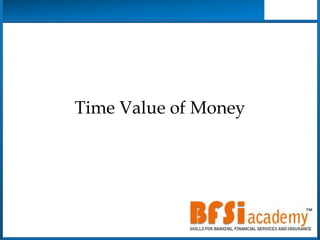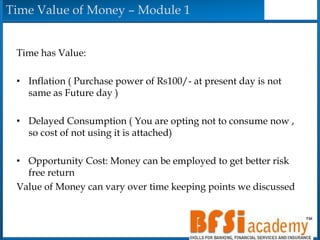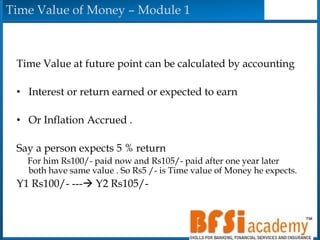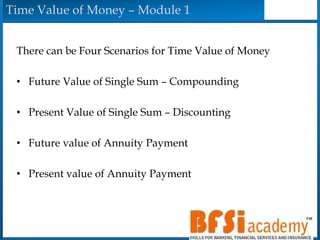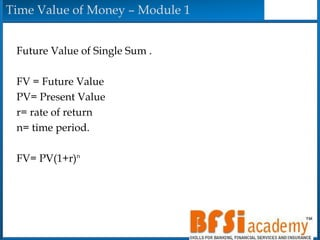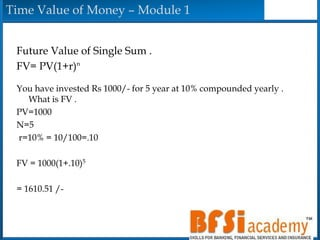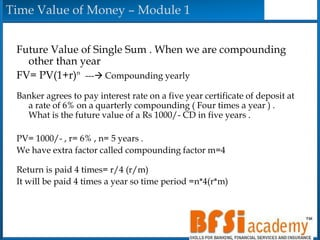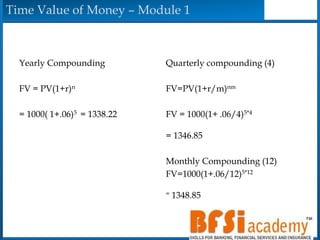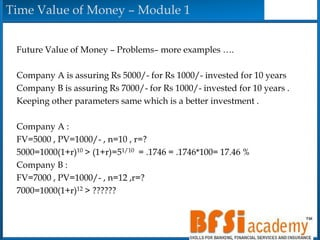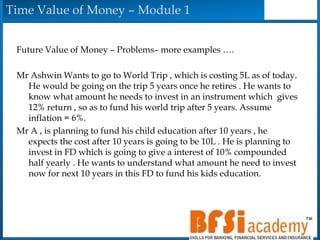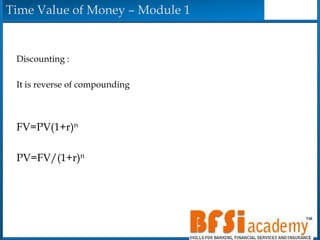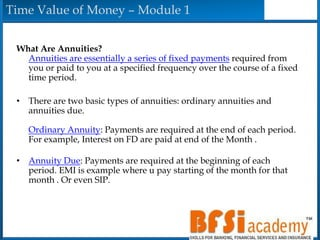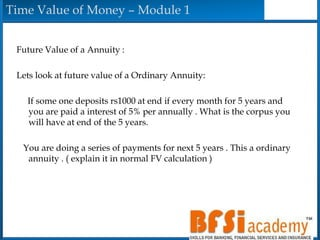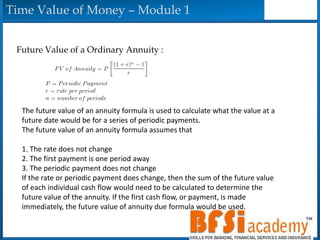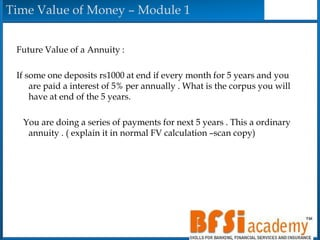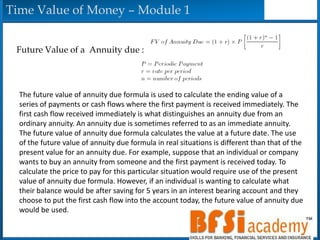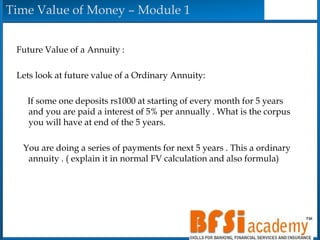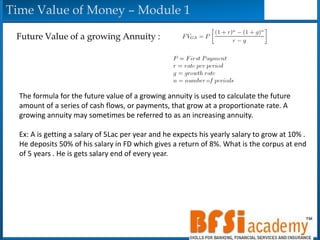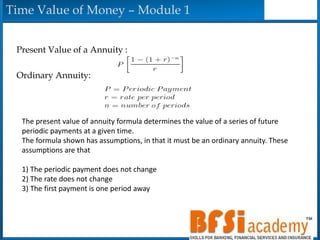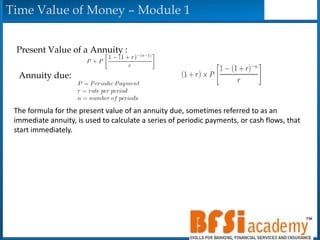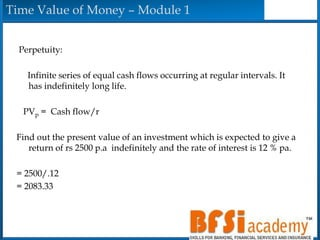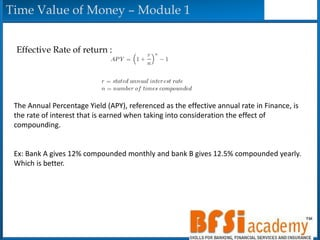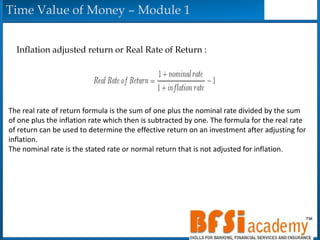1 de 23

### Time value of money

• 2. Time Value of Money – Module 1 Time has Value: • Inflation ( Purchase power of Rs100/- at present day is not same as Future day ) • Delayed Consumption ( You are opting not to consume now , so cost of not using it is attached) • Opportunity Cost: Money can be employed to get better risk free return Value of Money can vary over time keeping points we discussed
• 3. Time Value of Money – Module 1 Time Value at future point can be calculated by accounting • Interest or return earned or expected to earn • Or Inflation Accrued . Say a person expects 5 % return For him Rs100/- paid now and Rs105/- paid after one year later both have same value . So Rs5 /- is Time value of Money he expects. Y1 Rs100/- --- Y2 Rs105/-
• 4. Time Value of Money – Module 1 There can be Four Scenarios for Time Value of Money • Future Value of Single Sum – Compounding • Present Value of Single Sum – Discounting • Future value of Annuity Payment • Present value of Annuity Payment
• 5. Time Value of Money – Module 1 Future Value of Single Sum . FV = Future Value PV= Present Value r= rate of return n= time period. FV= PV(1+r)n
• 6. Time Value of Money – Module 1 Future Value of Single Sum . FV= PV(1+r)n You have invested Rs 1000/- for 5 year at 10% compounded yearly . What is FV . PV=1000 N=5 r=10% = 10/100=.10 FV = 1000(1+.10)5 = 1610.51 /-
• 7. Time Value of Money – Module 1 Future Value of Single Sum . When we are compounding other than year FV= PV(1+r)n --- Compounding yearly Banker agrees to pay interest rate on a five year certificate of deposit at a rate of 6% on a quarterly compounding ( Four times a year ) . What is the future value of a Rs 1000/- CD in five years . PV= 1000/- , r= 6% , n= 5 years . We have extra factor called compounding factor m=4 Return is paid 4 times= r/4 (r/m) It will be paid 4 times a year so time period =n*4(r*m)
• 8. Time Value of Money – Module 1 Yearly Compounding FV = PV(1+r)n = 1000( 1+.06)5 = 1338.22 Quarterly compounding (4) FV=PV(1+r/m)nm FV = 1000(1+ .06/4)5*4 = 1346.85 Monthly Compounding (12) FV=1000(1+.06/12)5*12 = 1348.85
• 9. Time Value of Money – Module 1 Future Value of Money – Problems– more examples …. Company A is assuring Rs 5000/- for Rs 1000/- invested for 10 years Company B is assuring Rs 7000/- for Rs 1000/- invested for 10 years . Keeping other parameters same which is a better investment . Company A : FV=5000 , PV=1000/- , n=10 , r=? 5000=1000(1+r)10 > (1+r)=51/10 = .1746 = .1746*100= 17.46 % Company B : FV=7000 , PV=1000/- , n=12 ,r=? 7000=1000(1+r)12 > ??????
• 10. Time Value of Money – Module 1 Future Value of Money – Problems– more examples …. Mr Ashwin Wants to go to World Trip , which is costing 5L as of today. He would be going on the trip 5 years once he retires . He wants to know what amount he needs to invest in an instrument which gives 12% return , so as to fund his world trip after 5 years. Assume inflation = 6%. Mr A , is planning to fund his child education after 10 years , he expects the cost after 10 years is going to be 10L . He is planning to invest in FD which is going to give a interest of 10% compounded half yearly . He wants to understand what amount he need to invest now for next 10 years in this FD to fund his kids education.
• 11. Time Value of Money – Module 1 Discounting : It is reverse of compounding FV=PV(1+r)n PV=FV/(1+r)n
• 12. Time Value of Money – Module 1 What Are Annuities? Annuities are essentially a series of fixed payments required from you or paid to you at a specified frequency over the course of a fixed time period. • There are two basic types of annuities: ordinary annuities and annuities due. Ordinary Annuity: Payments are required at the end of each period. For example, Interest on FD are paid at end of the Month . • Annuity Due: Payments are required at the beginning of each period. EMI is example where u pay starting of the month for that month . Or even SIP.
• 13. Time Value of Money – Module 1 Future Value of a Annuity : Lets look at future value of a Ordinary Annuity: If some one deposits rs1000 at end if every month for 5 years and you are paid a interest of 5% per annually . What is the corpus you will have at end of the 5 years. You are doing a series of payments for next 5 years . This a ordinary annuity . ( explain it in normal FV calculation )
• 14. Time Value of Money – Module 1 Future Value of a Ordinary Annuity : The future value of an annuity formula is used to calculate what the value at a future date would be for a series of periodic payments. The future value of an annuity formula assumes that 1. The rate does not change 2. The first payment is one period away 3. The periodic payment does not change If the rate or periodic payment does change, then the sum of the future value of each individual cash flow would need to be calculated to determine the future value of the annuity. If the first cash flow, or payment, is made immediately, the future value of annuity due formula would be used.
• 15. Time Value of Money – Module 1 Future Value of a Annuity : If some one deposits rs1000 at end if every month for 5 years and you are paid a interest of 5% per annually . What is the corpus you will have at end of the 5 years. You are doing a series of payments for next 5 years . This a ordinary annuity . ( explain it in normal FV calculation –scan copy)
• 16. Time Value of Money – Module 1 Future Value of a Annuity due : The future value of annuity due formula is used to calculate the ending value of a series of payments or cash flows where the first payment is received immediately. The first cash flow received immediately is what distinguishes an annuity due from an ordinary annuity. An annuity due is sometimes referred to as an immediate annuity. The future value of annuity due formula calculates the value at a future date. The use of the future value of annuity due formula in real situations is different than that of the present value for an annuity due. For example, suppose that an individual or company wants to buy an annuity from someone and the first payment is received today. To calculate the price to pay for this particular situation would require use of the present value of annuity due formula. However, if an individual is wanting to calculate what their balance would be after saving for 5 years in an interest bearing account and they choose to put the first cash flow into the account today, the future value of annuity due would be used.
• 17. Time Value of Money – Module 1 Future Value of a Annuity : Lets look at future value of a Ordinary Annuity: If some one deposits rs1000 at starting of every month for 5 years and you are paid a interest of 5% per annually . What is the corpus you will have at end of the 5 years. You are doing a series of payments for next 5 years . This a ordinary annuity . ( explain it in normal FV calculation and also formula)
• 18. Time Value of Money – Module 1 Future Value of a growing Annuity : The formula for the future value of a growing annuity is used to calculate the future amount of a series of cash flows, or payments, that grow at a proportionate rate. A growing annuity may sometimes be referred to as an increasing annuity. Ex: A is getting a salary of 5Lac per year and he expects his yearly salary to grow at 10% . He deposits 50% of his salary in FD which gives a return of 8%. What is the corpus at end of 5 years . He is gets salary end of every year.
• 19. Time Value of Money – Module 1 Present Value of a Annuity : Ordinary Annuity: The present value of annuity formula determines the value of a series of future periodic payments at a given time. The formula shown has assumptions, in that it must be an ordinary annuity. These assumptions are that 1) The periodic payment does not change 2) The rate does not change 3) The first payment is one period away
• 20. Time Value of Money – Module 1 Present Value of a Annuity : Annuity due: The formula for the present value of an annuity due, sometimes referred to as an immediate annuity, is used to calculate a series of periodic payments, or cash flows, that start immediately.
• 21. Time Value of Money – Module 1 Perpetuity: Infinite series of equal cash flows occurring at regular intervals. It has indefinitely long life. PVp = Cash flow/r Find out the present value of an investment which is expected to give a return of rs 2500 p.a indefinitely and the rate of interest is 12 % pa. = 2500/.12 = 2083.33
• 22. Time Value of Money – Module 1 Effective Rate of return : The Annual Percentage Yield (APY), referenced as the effective annual rate in Finance, is the rate of interest that is earned when taking into consideration the effect of compounding. Ex: Bank A gives 12% compounded monthly and bank B gives 12.5% compounded yearly. Which is better.
• 23. Time Value of Money – Module 1 Inflation adjusted return or Real Rate of Return : The real rate of return formula is the sum of one plus the nominal rate divided by the sum of one plus the inflation rate which then is subtracted by one. The formula for the real rate of return can be used to determine the effective return on an investment after adjusting for inflation. The nominal rate is the stated rate or normal return that is not adjusted for inflation.